Close Packing In Crystals & Radius Ratio Rules

# Close Packing In Crystals & Radius Ratio Rules Notes | Study Physical Chemistry - Chemistry

## Document Description: Close Packing In Crystals & Radius Ratio Rules for Chemistry 2022 is part of Physical Chemistry preparation. The notes and questions for Close Packing In Crystals & Radius Ratio Rules have been prepared according to the Chemistry exam syllabus. Information about Close Packing In Crystals & Radius Ratio Rules covers topics like Close packing in Crystals, Radius Ratio Rules: and Close Packing In Crystals & Radius Ratio Rules Example, for Chemistry 2022 Exam. Find important definitions, questions, notes, meanings, examples, exercises and tests below for Close Packing In Crystals & Radius Ratio Rules.

Introduction of Close Packing In Crystals & Radius Ratio Rules in English is available as part of our Physical Chemistry for Chemistry & Close Packing In Crystals & Radius Ratio Rules in Hindi for Physical Chemistry course. Download more important topics related with notes, lectures and mock test series for Chemistry Exam by signing up for free. Chemistry: Close Packing In Crystals & Radius Ratio Rules Notes | Study Physical Chemistry - Chemistry
 1 Crore+ students have signed up on EduRev. Have you?

Close packing in Crystals

(i) Close packing in two dimension: There are two ways to build a crystal plane

(a) Spheres are packed in a such a way that the rows have a horizontal as well as vertical alignment. In this arrangement, the spheres are found to form square. This type of packing is also called square close packing.
The number of spheres which are touching a given sphere is called the co-ordination number. Thus, the coordination number of each sphere in (a) is four.

(b) The spheres are packed in such a way t hat the spheres in the second row are placed in the depressions between the spheres of the first row and so on. This gives rise to hexagonal close packing of spheres and the coordination number of each sphere is six.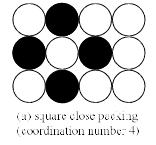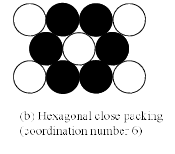(ii) Close packing in three dimensions: It is clear from the figure (a) that there are two types of voids or hollows in the first layer. These are marked as b and c. All the hollows are equivalent but the sphere of second layer may be placed either on hollows which are marked b or on the other set of hollows marked c. It may be noted that it is not possible to place spheres on both types of hollows so the second la yer is indicated as dotted in circles in figure b.

When a third layer is to be added, again there two types of hollows available. One type of hollows marked ‘a’ are unoccupied hollows of the first layer. The other types of hollows are hollows in the second layer (marked c). Thus, there are two alternatives to build the layer.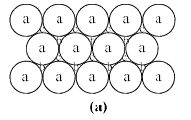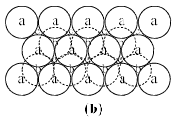(i) When the third layer is placed over the second layer so as to cover the tetrahedral or ‘c’ voids, a three – dimensional closest packing is obtained where t he spheres in ever y third layer are vertically aligned to the first layer. This arrangement is called ABAB......... pattern or hexagonal (HCP) close packing (calling first layer as A and second layer B).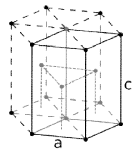(a) For HCP geometry Coordination number = 12 (b) For HCP geometry no. Of atoms per unit cell (corner)

= 12 (corner)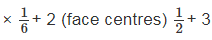(inside the body) ∗ 1 = 6

(c) For HCP geometry packing efficiency  = 74%

(ii) When the third layer is placed over the second layer such that the spheres cover the octahedral or ‘d’ voids, a layer c different from A and B is formed. This pattern is called ABCABC ......... pattern or cubic close packing (CCP). The ABCABC...... peaking has cubic symmetry and is known as cubic close packing (ccp). The cubic close packing has face centred (fcc) unit cell.

(a) For CCP geometry coordination number = 12

(b) For CCP geometry number of atoms per unit cell = 4 (as calculated before)

(c) For CCP geometry packing efficiency = 74% (as calculated before) In the close packing of spheres, certain hollows are left vacant. These holes or voids in the crystals are called interstitial sites or interstitial voids.

Two important interstitial sites are

(i) Triangular

(ii) Tetrahedral

(iii) Octahedral

(iv) Cubical void

In ionic crystals, the coordination numbers as well as the geometrical shapes of the crystals depend mainly on the relative sizes of the ions. The ration of the radii of the positive and negative ions is called radius ratio.

Radius ratio =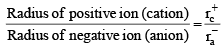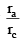Common coordination numbers are 3, 4, 6 and 8.

 Limiting ra/rc   radius ratio Co-ord. No. Shape Example < 0.155 2 Linear BeF2 0.155 – 0.225 3 Trigonal planar B2O3 0.225 – 0.414 4 Tetrahedral ZnS 0.414 – 0.732 4 Square planar PtCl4-2 0.414 – 0.732 6 Octahedral NaCl 0.732 – 0.999 8 B.C.C. CsCl
The document Close Packing In Crystals & Radius Ratio Rules Notes | Study Physical Chemistry - Chemistry is a part of the Chemistry Course Physical Chemistry.
All you need of Chemistry at this link: Chemistry

## Physical Chemistry

67 videos|106 docs|31 tests
 Use Code STAYHOME200 and get INR 200 additional OFF

## Physical Chemistry

67 videos|106 docs|31 tests

Track your progress, build streaks, highlight & save important lessons and more!

,

,

,

,

,

,

,

,

,

,

,

,

,

,

,

,

,

,

,

,

,

;